2018-11-08 17:09:35 qq_41682922 阅读数 122
• ###### 机器学习之降维与度量学习视频精讲

降维和度量学习是机器学习中的很重要的模块。降维，高纬度数据转化为低纬度的数据，主要是属性变化。本课程主要讲解：k近邻学习器、低维嵌入、主成成分分析、核化线性降维、流行学习、度量学习。

735 人正在学习 去看看 王而川

**

## 机器学习之入门级算法K近邻

**

K近邻算法与逻辑回归不同的是: K近邻可以解决多分类问题，而逻辑回归是解决二分类问题的（但是工作当中逻辑回归用的还是比较多的）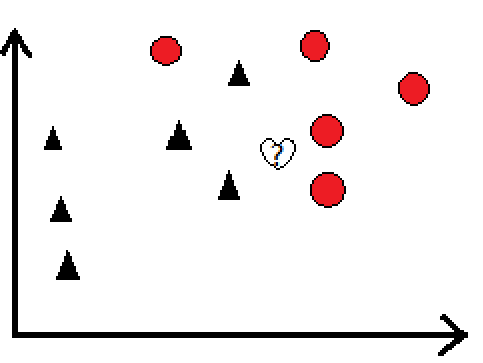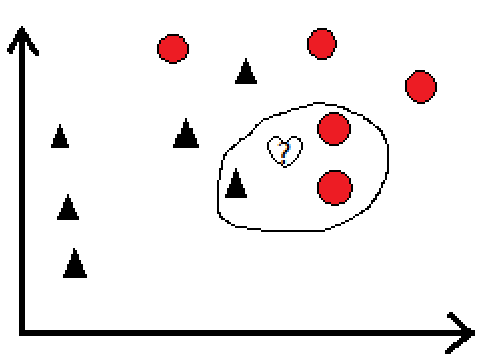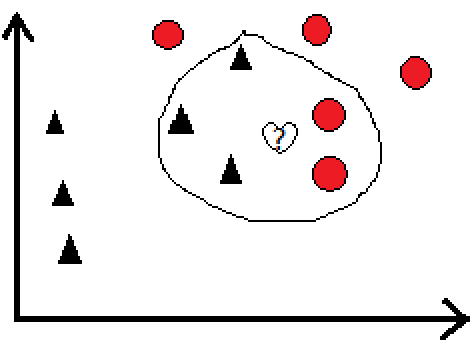• 计算测试数据与各个训练数据之间的距离
• 按照距离的递增关系进行排序
• 选取距离最小的k个点
• 确定前k个点所在类别的出现频率
• 返回前k个点钟出现频率最高的类别作为测试数据的预测分类

							  **原创**


k近邻机器学习 相关内容

2017-09-10 20:35:23 justry24 阅读数 591
• ###### 机器学习之降维与度量学习视频精讲

降维和度量学习是机器学习中的很重要的模块。降维，高纬度数据转化为低纬度的数据，主要是属性变化。本课程主要讲解：k近邻学习器、低维嵌入、主成成分分析、核化线性降维、流行学习、度量学习。

735 人正在学习 去看看 王而川

KNN算法没有显式的学习过程，事实上，它是“懒惰学习”的代表：在训练阶段仅仅是把样本保存起来，训练时间开销为0

（平均法）：
import numpy as np

def knnclassify(inX, dataSet, labels, k):
dataSetSize = dataSet.shape
diffMat = np.tile(inX, (dataSetSize,1)) - dataSet
sqDiffMat = diffMat**2
sqDistances = sqDiffMat.sum(axis=1)
distances = sqDistances**0.5
sortedDistIndicies = distances.argsort()
classCount = {}
for i in range(k):
voteIlabel = labels[sortedDistIndicies[i]]
classCount[voteIlabel] = classCount.get(voteIlabel, 0) + 1
sortedClassCount = sorted(classCount.items(), key=lambda classCount : classCount, reverse=True)
return sortedClassCount

（加权投票法）

classCount[votelabel] = classCount.get(votelabel, 0) + round((1.5 - i / (k-1)), 2)

 k 错误总数（平均投票） 错误总数（加权投票） 1 13 13 2 13 13 3 10 13 4 11 10 5 17 11 6 17 14 7 21 18 8 18 16 9 21 19

1. 并不是 K 越大，分类准确率越高。
2. 当 K 越大时，加权投票的分类 优势越明显

import numpy as np
from knn.KNNclassifier import knnclassify
# 导入当前文件夹下的文件，(如果不加入"knn.",则会出现红色波浪线，但可正常运行）
def creatrDateSet():
group = np.array([[1.0,1.1],[1.0,1.0],[0,0],[0,0.1]])
labels = ['A','A','B','B']
return group, labels

group, labels = creatrDateSet()
print(knnclassify([0, 0], group, labels, 3))  # 结果B
print(knnclassify([1, 1], group, labels, 4))  # 结果A
print(knnclassify([0.5, 0.5], group, labels, 4))  # 结果B

def classify0(inX, dataSet, labels, k):
dataSetSize = dataSet.shape #4
diffMat = np.tile(inX, (dataSetSize,1)) - dataSet
#  np.tile(inX, (dataSetSize,1) 结果为 [[0,0],[0,0],[0,0],[0,0]]
# 减去dataSet 后每行为每个点的距离差
sqDiffMat = diffMat**2
# 将每个距离差平方
sqDistances = sqDiffMat.sum(axis=1)
# #axis=0表述列 ，axis=1表述行, 横项相加
distances = sqDistances**0.5

# 开根号得到距离:[ 1.48660687  1.41421356  0.          0.1      ]
sortedDistIndicies = distances.argsort() # 返回结果从头到尾为非降序排序的index：[2 3 1 0]

classCount={}
for i in range(k):
voteIlabel = labels[sortedDistIndicies[i]]
# 循环取出排序从近到远的labels  : B B A
classCount[voteIlabel] = classCount.get(voteIlabel,0) + 1 # 构建字典，最终得到频次{'B': 2, 'A': 1}

sortedClassCount = sorted(classCount.items(), key= lambda classCount:classCount, reverse=True)
# operator模块提供的itemgetter函数用于获取对象的哪些维的数据
# sortedClassCount = sorted(classCount.iteritems(), key=operator.itemgetter(1), reverse=True)
'''在Python2.x中，items( )用于 返回一个字典的拷贝列表【Returns a copy of the list of all items (key/value pairs) in D】，占额外的内存。
iteritems() 用于返回本身字典列表操作后的迭代【Returns an iterator on all items(key/value pairs) in D】，不占用额外的内存。
Python 3.x 里面，iteritems() 和 viewitems() 这两个方法都已经废除了，而 items() 得到的结果是和 2.x 里面 viewitems() 一致的。
在3.x 里 用 items()替换iteritems() ，以列表返回可遍历的(键, 值) 元组数组'''

print(sortedClassCount)
return sortedClassCount
# sortedClassCount 得到的值为列表，列表元素为每个标签和频次，由频次从高到底排序 ，本例中为[('B', 2), ('A', 1)]

group, labels = creatrDateSet()
print(classify0([0, 0], group, labels, 3))  # 结果B
print(classify0([1, 1], group, labels, 4))  # 结果A
print(classify0([0.5, 0.5], group, labels, 4))  # 结果B

import numpy as np
from knn.KNNclassifier import knnclassify

# 将 文本记录 转换为 NumPy
def file2matrix(filename, dim):
fr = open(filename)
numberOfLines = len(fr.readlines())        #get the number of lines in the file
returnMat = np.zeros((numberOfLines,dim))        #prepare matrix to return
classLabelVector = []                      #prepare labels return
fr = open(filename)
index = 0
line = line.strip()
listFromLine = line.split('\t')
returnMat[index,:] = listFromLine[0:dim]
classLabelVector.append(int(listFromLine[-1]))
index += 1
return returnMat,classLabelVector

def normalize(dataSet):
minVals = dataSet.min(0) # 每列最小值
maxVals = dataSet.max(0)
ranges = maxVals - minVals
normDataSet = np.zeros(np.shape(dataSet))
m = dataSet.shape
normDataSet = dataSet - np.tile(minVals, (m, 1))
normDataSet = normDataSet/np.tile(ranges, (m, 1))
return normDataSet , ranges, minVals

def dataplot(dataSet, labels):
import  matplotlib.pyplot as plt
fig = plt.figure()
ax.scatter(dataSet[:,1], dataSet[:,2], 15.0*np.array(labels), np.array(labels))
ax.set_ylabel('Ice Cream Consumption')
ax.set_xlabel('Time of Playing Games')
# 后面两个参数分别控制散点大小和颜色
plt.show()

def classifytest(dataset, datalabel, k, testRatio):
m = dataset.shape # 数据列数
numTestVecs = int(m * testRatio) #此次测试所用的样本数目
errorCount = 0.0
for i in range(numTestVecs):
classifierResult = knnclassify(dataset[i, :], dataset[numTestVecs:m, :], datalabel[numTestVecs:m], k)
#print("the classifier came back with: %d, the real answer is: %d" % (classifierResult, datalabel[i]))
# dataset 前 测试率*数据总数目 用于测试，  使用 余下的作为分类依据
if (classifierResult != datalabel[i]):
errorCount += 1.0
print("the total error rate is: %f" % (errorCount / float(numTestVecs)))

def main():

datingDataMat, datingLabels = file2matrix('datingTestSet2.txt',3)
normMat, ranges, minVals = normalize(datingDataMat)
dataplot(normMat, datingLabels)
#classifytest(normMat,datingLabels,3,0.1) # 第三个参数为分类器的k值
# 第四个系数为测试样本比例，系数越高 分类准确率越低（用于测试的越多，用于分类的越少）

resultList = ['not at all', 'in small dose', 'in large doses']
timeofgame = float(input("percentage of time spend playing video games:"))
flymiles = float(input("frequent flier miles earner per year:"))
icecream = float(input("liters of ice cream consumed per year:"))
inarr = np.array([timeofgame, flymiles, icecream])
norminput = (inarr - minVals) / ranges
result = knnclassify(norminput, normMat, datingLabels, 3)
print("you will probable like this person", resultList[result - 1])

if __name__=="__main__":
main()

import numpy as np
from os import listdir
from knn.KNNclassifier import knnclassify

# 将 32*32 的数组，转化为一维 nd.array 矩阵
def img2vector(filename):
returnVect = np.zeros((1,1024))
fr = open(filename)
for i in range(32):
for j in range(32):
returnVect[0,32*i+j] = int(lineStr[j])
return returnVect

def handwritingClassTest():
hwLabels = []
trainingFileList = listdir('digits/trainingDigits')          #load the training set
m = len(trainingFileList)  # 文件数目，同时也是训练样本数目 本例中为1934
trainingMat = np.zeros((m,1024))
for i in range(m):
fileNameStr = trainingFileList[i]
fileStr = fileNameStr.split('.')    #take off .txt
classNumStr = int(fileStr.split('_'))
hwLabels.append(classNumStr)  # 得到训练样本的真实标签
trainingMat[i,:] = img2vector('digits/trainingDigits/%s' % fileNameStr)
# 对每个文件进行 向量化处理，得到 m * 1024 的矩阵

testFileList = listdir('digits/testDigits')        #iterate through the test set
errorCount = 0.0
mTest = len(testFileList)
for i in range(mTest):
fileNameStr = testFileList[i]
fileStr = fileNameStr.split('.')    # 去掉 .txt 的后缀
classNumStr = int(fileStr.split('_'))  # _ 前为该数字的正确值
vectorUnderTest = img2vector('digits/testDigits/%s' % fileNameStr)
classifierResult = knnclassify(vectorUnderTest, trainingMat, hwLabels, 3)  #对每个测试样本进行分类
#print("the classifier came back with: %d, the real answer is: %d" % (classifierResult, classNumStr))
if (classifierResult != classNumStr): errorCount += 1.0
print("\nthe total number of errors is: %d" % errorCount)
print("\nthe total error rate is: %f" % (errorCount/float(mTest)))

handwritingClassTest()
# 约会选择中，将一份数据一分为2位训练集和测试集，而此例中为分开好的
# 由于数据只能为 0 和 1， 因此无需归一化处理

k近邻机器学习 相关内容

2018-08-23 11:55:54 Arwen_H 阅读数 1710
• ###### 机器学习之降维与度量学习视频精讲

降维和度量学习是机器学习中的很重要的模块。降维，高纬度数据转化为低纬度的数据，主要是属性变化。本课程主要讲解：k近邻学习器、低维嵌入、主成成分分析、核化线性降维、流行学习、度量学习。

735 人正在学习 去看看 王而川

# k近邻介绍

——K最近邻(k-Nearest Neighbor，KNN)，k近邻算法可以应用于分类场景与回归场景，是一个理论上比较不成熟的方法，也是最简单的机器学习算法之一。该方法的思路是：如果一个样本在特征空间中的k个最相似(即特征空间中最邻近)的样本中的大多数属于某一个类别，则该样本也属于这个类别。
——用官方的话来说，所谓K近邻算法，即是给定一个训练数据集，对新的输入实例，在训练数据集中找到与该实例最邻近的K个实例（也就是上面所说的K个邻居）， 这K个实例的多数属于某个类，就把该输入实例分类到这个类中。

: 看图举例：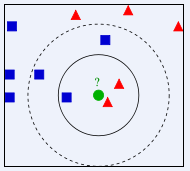K近邻在sklearn里面的API为sklearn.neighbors，在该模块下由细分了13个小的模块。本文主要使用neighbors.KNeighborsRegressor基于k-最近邻居的回归来进行说明官方教程链接

neighbors.BallTree BallTree用于快速广义N点问题
neighbors.DistanceMetric DistanceMetric类
neighbors.KDTree KDTree用于快速广义N点问题
neighbors.KernelDensity([bandwidth, …]) 核密度估计
neighbors.KNeighborsClassifier([…]) 实现k近邻的分类器投票
neighbors.KNeighborsRegressor([n_neighbors, …]) 基于k-最近邻居的回归
neighbors.LocalOutlierFactor([n_neighbors, …]) 使用局部异常因子（LOF）的无监督异常值检测
neighbors.NearestCentroid([metric, …]) 最近的质心分类器
neighbors.NearestNeighbors([n_neighbors, …]) 用于实现邻居搜索的无监督学习者
neighbors.kneighbors_graph(X, n_neighbors[, …]) 计算X中点的k-邻居的（加权）图

# k近邻参数

sklearn.neighbors.KNeighborsRegressor默认参数：

sklearn.neighbors.KNeighborsRegressor（n_neighbors = 5，weights =‘uniform’，algorithm =‘auto’，leaf_size = 30，p = 2，metric =‘minkowski’，metric_params = None，n_jobs = 1，** kwargs ）

n_neighbors：int, optional (default = 5)

weights : str or callable

‘uniform’ :统一的重量。权重分在每个社区都是一样的
‘distance’ :重量分距离的倒数。在这种情况下,接近邻居查询的点会有一个更大的影响力远比邻居
[callable] :一个用户定义的函数接受一个数组的距离,并返回一个数组包含权重相同的形状

algorithm : {‘auto’, ‘ball_tree’, ‘kd_tree’, ‘brute’}, optional

‘ball_tree’将使用BallTree
‘kd_tree’将使用KDTree
'brute’将使用蛮力搜索
‘auto’将试图确定最合适的算法基于价值观传递给合适的方法

leaf_size : int, optional (default = 30)

metric : string or callable, default ‘minkowski’

metric_params : dict, optional (default = None)

n_jobs : int, optional (default = 1)

## 官网例子

X=[,,,] #建一个List
y=[0,0,1,1] #建一个List
from sklearn.neighbors import KNeighborsRegressor #导入K近邻回归模块
neigh=KNeighborsRegressor(n_neighbors=2) #近邻参数设置成2个相邻
neigh.fit(X, y) #使用X作为训练数据，y作为目标值
print(neigh.predict([[1.5]])) #给提供的数据预测对应的标签，当x=1.5的时候y应该是多少

#最终输出当x=1.5的时候y=0.5


fit(X, y) 使用X作为训练数据，y作为目标值（类似于标签）来拟合模型。
get_params([deep]) 获取估值器的参数。
kneighbors([X, n_neighbors, return_distance]) 查找一个或几个点的K个邻居
kneighbors_graph([X, n_neighbors, mode]) 计算在X数组中每个点的k邻居的（权重）图
predict(X) 给提供的数据预测对应的标签
predict_proba(X) 返回测试数据X的概率估值
score(X, y[, sample_weight]) 返回给定测试数据和标签的平均准确值
set_params(**params) 设置估值器的参数

# k近邻实际应用

## 房租价格预测

import pandas as pd #导入pandas库
from sklearn.neighbors import KNeighborsRegressor #导入机器学习库中的K近邻回归模型
from sklearn.metrics import mean_squared_error #导入机器学习库中的均方误差回归损失模型


dc_listings=pd.read_csv(r'C:\Users\huangjunwen\Desktop\listings.csv') #将数据导入,路径请根据自己的文件路径设置
features = ['accommodates','bedrooms','bathrooms','beds','price','minimum_nights','maximum_nights','number_of_reviews'] #因数据太多设置只选取这8列
dc_listings = dc_listings[features] #获取只需要的数据列，并覆盖原始数据
dc_listings['price']=dc_listings.price.str.replace("\\$|,",'').astype(float) #将价格price变成数字类型
dc_listings=dc_listings.dropna() #过滤缺失数据
normalized_listings = dc_listings #复制一下数据

norm_train_df=normalized_listings.copy().iloc[0:2792] #创建训练集训练集取2792个样本
norm_test_df=normalized_listings.copy().iloc[2792:] #创建测试集取879个样本


cols = ['accommodates','bedrooms','bathrooms','beds','minimum_nights','maximum_nights','number_of_reviews'] #选择测试集的训练的列
knn = KNeighborsRegressor(10) #模型近邻值手动设置成10,其他为默认参数
knn.fit(norm_train_df[cols], norm_train_df['price']) #X放入训练集数据，Y放入目标输出数据
two_features_predictions = knn.predict(norm_test_df[cols]) #输出测试集结果


two_features_mse = mean_squared_error(norm_test_df['price'], two_features_predictions)
two_features_rmse = two_features_mse ** (1/2)
print(two_features_rmse) #输出模型验证结果，根据结果调整近邻参数


print(knn.predict([[6,3,3,3,1,30,0]])) #设置自己房子的信息，预测出对应的价格


k近邻机器学习 相关内容

2017-07-07 15:24:11 shenhuaifeng 阅读数 338
• ###### 机器学习之降维与度量学习视频精讲

降维和度量学习是机器学习中的很重要的模块。降维，高纬度数据转化为低纬度的数据，主要是属性变化。本课程主要讲解：k近邻学习器、低维嵌入、主成成分分析、核化线性降维、流行学习、度量学习。

735 人正在学习 去看看 王而川

## 1.k近邻思想

中国有古话”物理类聚,人以群分”,k近邻思想貌似也是这个意思.例如我想判断一个人是好人还是坏人,但是我对这个人根本不了解,那么我如何去判断呢?当然,如果不给我任何其它条件那么我只能靠”颜值”去判断了…,所以这里有个条件——-我虽然不认这个人,但我认识他所有的朋友并且知道他的每一个朋友是好人还是坏人.例如如他的10个朋友其中有8个朋友是坏人,2个是好人,那么我就可以判断他很可能是个坏人,反之亦然.
那么在数据集中又是如何体现的呢?假设给我们一堆已知类别的二维数据{(x0,y0),(x1,y1),(xn,yn)}$\{(x_0,y_0),(x_1,y_1)\cdots,(x_n,y_n)\}$并且已知数据一共分为三类z1,z2,z3$z_1,z_2,z_3$(实际情况可能是三维四维等更复杂的情况,但是原理应该都是一样的),然后给我一个(x,y)$(x,y)$让我去确定这个未知的(x,y)$(x,y)$的类别.那么我应该如何应用k近邻算法呢?
* 步骤1:计算(x,y)$(x,y)$与所有已知数据(xi,yi)$(x_i,y_i)$的欧式距离

di=(xxi)2+(yyi)2$d_i = \sqrt {(x-x_i)^2+(y-y_i)^2}$

*步骤2:选取距离(x,y)$(x,y)$最近的k个点
*步骤3:统计k个点中三个类别标签的个数
*步骤4:选取标签个数最多的类别作为(x,y)$(x,y)$的类别

## 2.k近邻算法的代码实现

### 2.1创建数据

'''

'''
def createDataSet():
group=array([[1.0,1.1],[1.0,1.0],[0,0],[0,0.1]])#这两行代码定义一组数据和数据对应的类别
labels=['A','A','B','B']
return group,labels

## 2.2k近邻算法

'''

'''
def classify0(inX,dataSet,labels,k):#inX:待分类数据,dataSet:数据集,labels:数据集对应标签,k指定距离inX最近数据点的个数
dataSetSize=dataSet.shape #获取数据个数
diffMat=tile(inX,(dataSetSize,1))-dataSet #x-x1,y-y1
sqDiffMat=diffMat**2              #(x-x1)^2
sqDistances=sqDiffMat.sum(axis=1) #
distances=sqDistances**0.5        #距离D
sorteDistIndicies=distances.argsort() #对距离从小到大排序
classCount={}
for i in range(k):  #统计前k个距离个各个类标签出现的次数
voteIlabel=labels[sorteDistIndicies[i]]
classCount[voteIlabel]=classCount.get(voteIlabel,0)+1
sortedClassCount=sorted(classCount.items(),key=operator.itemgetter(1),reverse=True)
return sortedClassCount#返回出现次数最多的类别标签

## 2.3对数据归一化

为什么要数据归一化呢?例如我们训练数据(0.1,100)$(0.1,100)$其中的第一个特征与第二个特征差别的别大,那么在计算距离

d=(x0.1)2+(y100)2$d=\sqrt{(x-0.1)^2+(y-100)^2}$

xnew=xxminxmaxxmin$x_{new}=\frac{x-x_{min}}{x_{max}-x_{min}}$

'''

'''
def autoNorm(dataSet):
minVals = dataSet.min(0) #min()参数0,找出每一列的最小值
maxVals = dataSet.max(0)
ranges = maxVals-minVals
normDataSet = zeros(shape(dataSet))
m=dataSet.shape
normDataSet=dataSet-tile(minVals,(m,1))#tile()把,minVlas,复制m行,1列
normDataSet =normDataSet/tile(ranges,(m,1))
return normDataSet,ranges,minVals

## 2.4综合测试

数据集在我的github上

'''

'''
def datingClassTest():
hoRatio=0.10        #训练数据集:测试数据集=1:9
datingDataMat,datingLabels=file2matrix('datingTestSet.txt')
normMat,ranges,minVals=autoNorm(datingDataMat) #正则化数据
m=normMat.shape                             #获取样本数量
numTestVecs=int(m*hoRatio)                     #获取测试样本数量
errorCount=0
for i in range(numTestVecs):
classifierResult=classify0(normMat[i,:],normMat[numTestVecs:m,:],datingLabels[numTestVecs:m],3)
print("the calssifier came back with:%d,the real answer is:%d" % (classifierResult,datingLabels[i]))
if (classifierResult != datingLabels[i]):
errorCount +=1
print("the total error rate is: %f" %(errorCount/float(numTestVecs)))

在python3命令行模式输入

import kNN
kNN.datingClassTest()k近邻机器学习 相关内容

2019-02-26 19:49:00 qq_37960402 阅读数 62
• ###### 机器学习之降维与度量学习视频精讲

降维和度量学习是机器学习中的很重要的模块。降维，高纬度数据转化为低纬度的数据，主要是属性变化。本课程主要讲解：k近邻学习器、低维嵌入、主成成分分析、核化线性降维、流行学习、度量学习。

735 人正在学习 去看看 王而川

k近邻算法：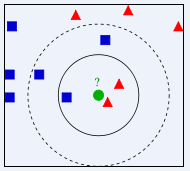k=3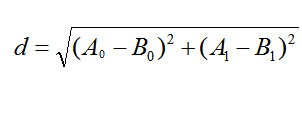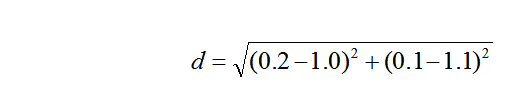1、简单，易于理解，易于实现，无需估计参数，无需训练
2、适合对稀有事件进行分类
3、特别适合于多分类问题(multi-modal,对象具有多个类别标签)， kNN比SVM的表现要好

1、该算法在分类时有个主要的不足是，当样本不平衡时，如一个类的样本容量很大，而其他类样本容量很小时，有可能导致当输入一个新样本时，该样本的K个邻居中大容量类的样本占多数。 该算法只计算“最近的”邻居样本，某一类的样本数量很大，那么或者这类样本并不接近目标样本，或者这类样本很靠近目标样本。无论怎样，数量并不能影响运行结果。
2、该方法的另一个不足之处是计算量较大，因为对每一个待分类的文本都要计算它到全体已知样本的距离，才能求得它的K个最近邻点。

from numpy import *
import operator
def createDataSet():    #创建标签和数据
group=array([[1.0,1.1],[1.0,1.0],[0,0],[0,0.1]])
labels=['A','A','B','B']
return group,labels
def classifify0(inX,dataSet,labels,k):
dataSetSize=dataSet.shape      # dataSetSize=4    计算距离
diffMat=tile(inX,(dataSetSize,1))-dataSet #tile(inX,(dataSetSize,1)),让inX变为和dataSet一样的类型
sqDiffMat=diffMat**2 #
sqDistances=sqDiffMat.sum(axis=1)
distances=sqDistances**0.5
sortedDistIndicies=distances.argsort()
classCount={}

for i in range(k):         #选择距离最小的k个节点
voteIlabel=labels[sortedDistIndicies[i]]
classCount[voteIlabel]=classCount.get(voteIlabel,0)+1
sortedCalssCount=sorted(classCount.items(),
key=operator.itemgetter(1),reverse=True)
return sortedCalssCount
group,labels=createDataSet()
print(classifify0([1, 0.5], group, labels, 3))


from numpy import *
import operator
from keras.datasets import mnist
import numpy as np
X_train=X_train[0:10000]        #取10000个训练
Y_train=Y_train[0:10000]
X_test=X_test[0:100]             #取100个测试
Y_test=Y_test[0:100]
aa=X_train.reshape(1,784)
def classifify0(inX,dataSet,labels,k):
dataSetSize=dataSet.shape      # dataSetSize=4
diffMat=tile(inX,(dataSetSize,1))-dataSet #tile(inX,(dataSetSize,1)),让inX变为和dataSet一样的类型
sqDiffMat=diffMat**2 #
sqDistances=sqDiffMat.sum(axis=1)
distances=sqDistances**0.5
sortedDistIndicies=distances.argsort()
classCount={}
for i in range(k):
voteIlabel=labels[sortedDistIndicies[i]]
classCount[voteIlabel]=classCount.get(voteIlabel,0)+1
sortedCalssCount=sorted(classCount.items(),
key=operator.itemgetter(1),reverse=True)
return sortedCalssCount

def handwriting(X_train,Y_train,X_test,k):
hwlabels=[]
m=len(X_train)
trainingMat=zeros((m,784))
for i in range(m):
trainingMat[i,:]=X_train[i].reshape(1,784)   #将图片转换为向量形式
mTest=len(X_test)
for i in range(mTest):
wordtest=X_test[i].reshape(1,784)
classresult=classifify0(wordtest,trainingMat,Y_train,k)
hwlabels.append(classresult)
#print("result:{},true{}".format(classresult,Y_test[i]))
return hwlabels
k=[10,50,100,150]         #取前k个
for i in range(len(k)):
classresult=handwriting(X_train,Y_train,X_test,k[i])
aa=(classresult == Y_test).astype(np.int32)
acc=sum(aa)/len(Y_test)
print(acc)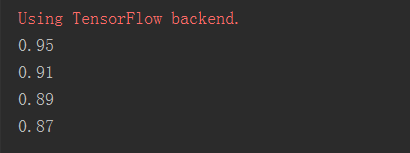k近邻机器学习 相关内容